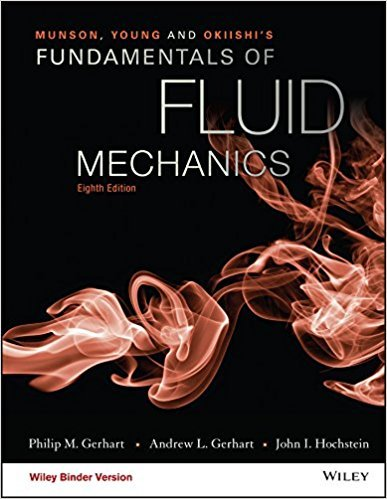×
×

# Determine the height that water at 60 F will rise due tocapillary action in a cleanISBN: 9781119080701 456

## Solution for problem 1.129 Chapter 1.9

Fundamentals of Fluid Mechanics | 8th Edition

• Textbook Solutions
• 2901 Step-by-step solutions solved by professors and subject experts
• Get 24/7 help from StudySoup virtual teaching assistantsFundamentals of Fluid Mechanics | 8th Edition

4 5 1 365 Reviews
21
4
Problem 1.129

Determine the height that water at 60 F will rise due tocapillary action in a clean, 14-in.-diameter tube (see Video V1.10).What will be the height if the diameter is reduced to 0.01 in.?

Step-by-Step Solution:
Step 1 of 3

G l O" C,l\na.*r {-€If **U.r\$ab.,uri tl{_.*.\'r,,i G U-\' f ,rbrcitV\ tJ.-,.krr"a f*n-,u-,^{-ifvt^,lo{ C ActcLoro-hqln ua,,r;Grr,1...

Step 2 of 3

Step 3 of 3

##### ISBN: 9781119080701

The full step-by-step solution to problem: 1.129 from chapter: 1.9 was answered by , our top Science solution expert on 03/16/18, 03:21PM. This full solution covers the following key subjects: . This expansive textbook survival guide covers 112 chapters, and 1357 solutions. Fundamentals of Fluid Mechanics was written by and is associated to the ISBN: 9781119080701. The answer to “Determine the height that water at 60 F will rise due tocapillary action in a clean, 14-in.-diameter tube (see Video V1.10).What will be the height if the diameter is reduced to 0.01 in.?” is broken down into a number of easy to follow steps, and 33 words. This textbook survival guide was created for the textbook: Fundamentals of Fluid Mechanics, edition: 8. Since the solution to 1.129 from 1.9 chapter was answered, more than 219 students have viewed the full step-by-step answer.

Unlock Textbook Solution